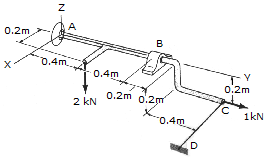# Engineering Mechanics - Equilibrium of a Rigid Body - Discussion

### Discussion :: Equilibrium of a Rigid Body - General Questions (Q.No.6)

6.There is a ball and socket connection at A. At point B there is a connection that opposes motion in the x and z directions only. Determine the unknown force components at A and B. Use a scalar analysis.

 [A]. Ax = 5.5 kN, Ay = -1.0 kN, Az = 2.75 kN, Bx = 3.5 kN, Bz = 1.25 kN, FDC = 2.0 kN [B]. Ax = 1.5 kN, Ay = -1.0 kN, Az = 0.75 kN, Bx = -3.5 kN, Bz = 1.25 kN, FDC = 2.0 kN [C]. Ax = 5.5 kN, Ay = -1.0 kN, Az = 2.75 kN, Bx = 3.5 kN, Bz = -0.75 kN, FDC = 2.0 kN [D]. Ax = 1.5 kN, Ay = -1.0 kN, Az = 1.25 kN, Bx = -3.5 kN, Bz = 0.75 kN, FDC = 2.0 kN

Explanation:

No answer description available for this question.

 Dhanraj Patil said: (Oct 12, 2011) Option D is correct.